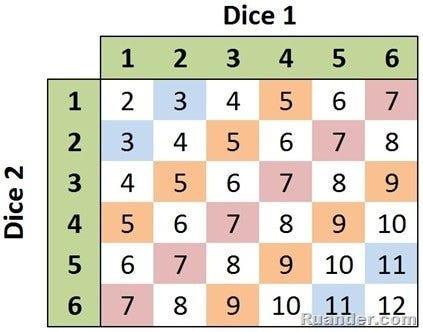# Introduction to Probability

Original article was published on Artificial Intelligence on Medium# Introduction to Probability

“It is likely that unlikely things should happen.” — Aristotle

Every year, we would organize a fun-fair in high school, as a day off to chill while getting some first-hand learning exposure, as the students were the organizers, the charm and the business model of the exhibition. We used to put up booths with a “pay-to-play” scheme of games and try to trick people into an unfair play (which didn’t work).

I remember taking part in one such game called ‘7-up/7-down’. The rules were straightforward — the host rolls two dies and you have to bet beforehand whether the sum of the face values of those dies would be greater or less than 7. If your prediction was correct, you win X times (say twice) the money you bet on, if incorrect, you lose the wagered money, and if the sum was exactly 7, you get back your gambled money (break-even). It was a fair game as the probability of getting a sum greater than 7 is the same as the probability of getting a sum less than 7.

P (>7) = 15/36

P (<7) = 15/36

P (=7) = 6/36

Let’s look at how we arrived at these numbers:

As seen from Fig 1, there are 36 outcomes for a sum of two dies [sum (1,1),…, sum (6,6)]. Total combinations with a sum greater than 7 are 15, combinations with sum less than 7 are also 15, and there are 6 combinations where the sum is exactly 7 (the purple diagonal). Hence, the probability of getting a sum greater than 7 is 15/36 (similarly for less than 7) and the probability of getting a sum equal to 7 is 6/36.

But you probably knew that much already (check references below if you didn’t). This essay tackles the question — “what it means to get a probability of 41.67% (15/36)”?

# An Experiment

Let’s flip a (fair) coin 10 times and record the face values.